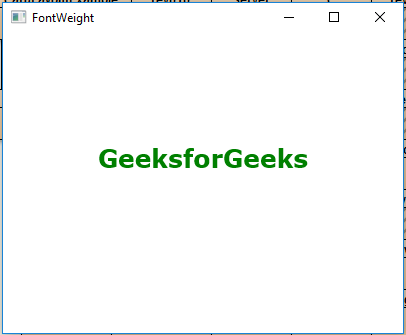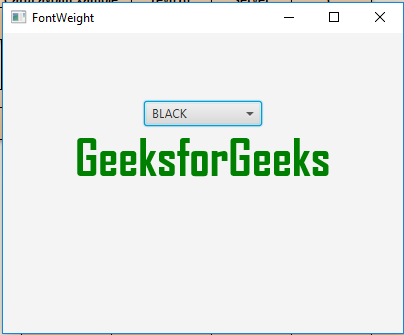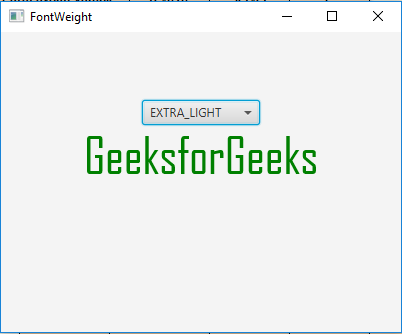# JavaFX | FontWeight Class

FontWeight class is a part of JavaFX. FontWeight class defines the weight of the font. FontWeight class specifies different font weights which can be used when searching for a font on the system. FontWeight class inherits Enum class.

Commonly Used Methods:

Method Explanation
findByName(String n) Returns FontWeight by its name.
findByWeight(int w) Returns the closest FontWeight for a weight value.
getWeight() Return the visual weight.
valueOf(String n) Returns the enum constant of this type with the specified name.
values() returns all the values of the FontWeight type.

Below programs illustrate the use of FontWeight Class:

1. Java program to create a TextFlow and add text object to it, set text Alignment and also set font weight of the font of the text and set line spacing of the text flow: In this program we will create a TilePane named tile_pane. Add Label named label and some buttons to the tile_pane. Set the Alignment of the tile_pane using the setAlignment() function. Set the Font weight of the font to EXTRA_BOLD. Add the tile_pane to the scene and add the scene to the stage and call the show() function to display the final results.

 `// Java program to create a TextFlow and ` `// add text object to it, set text Alignment ` `// and also set font weight of the font of text ` `// and set line spacing of the text flow. ` `import` `javafx.application.Application; ` `import` `javafx.scene.Scene; ` `import` `javafx.scene.control.*; ` `import` `javafx.scene.layout.*; ` `import` `javafx.stage.Stage; ` `import` `javafx.scene.layout.*; ` `import` `javafx.scene.paint.*; ` `import` `javafx.scene.text.*; ` `import` `javafx.geometry.*; ` `import` `javafx.scene.layout.*; ` `import` `javafx.scene.shape.*; ` ` `  `public` `class` `FontWeight_1 ``extends` `Application { ` ` `  `    ``// launch the application ` `    ``public` `void` `start(Stage stage) ` `    ``{ ` ` `  `        ``try` `{ ` ` `  `            ``// set title for the stage ` `            ``stage.setTitle(``"FontWeight"``); ` ` `  `            ``// create TextFlow ` `            ``TextFlow text_flow = ``new` `TextFlow(); ` ` `  `            ``// create text ` `            ``Text text_1 = ``new` `Text(``"GeeksforGeeks\n"``); ` ` `  `            ``// set the text color ` `            ``text_1.setFill(Color.GREEN); ` ` `  `            ``// set font of the text ` `            ``text_1.setFont(Font.font(``"Verdana"``, FontWeight.EXTRA_BOLD, ``25``)); ` ` `  `            ``// set text ` `            ``text_flow.getChildren().add(text_1); ` ` `  `            ``// set text Alignment ` `            ``text_flow.setTextAlignment(TextAlignment.CENTER); ` ` `  `            ``// set line spacing ` `            ``text_flow.setLineSpacing(``20``.0f); ` ` `  `            ``// create VBox ` `            ``VBox vbox = ``new` `VBox(text_flow); ` ` `  `            ``// set alignment of vbox ` `            ``vbox.setAlignment(Pos.CENTER); ` ` `  `            ``// create a scene ` `            ``Scene scene = ``new` `Scene(vbox, ``400``, ``300``); ` ` `  `            ``// set the scene ` `            ``stage.setScene(scene); ` ` `  `            ``stage.show(); ` `        ``} ` ` `  `        ``catch` `(Exception e) { ` ` `  `            ``System.out.println(e.getMessage()); ` `        ``} ` `    ``} ` ` `  `    ``// Main Method ` `    ``public` `static` `void` `main(String args[]) ` `    ``{ ` ` `  `        ``// launch the application ` `        ``launch(args); ` `    ``} ` `} `

Output:2. Java program to create a TextFlow and add text object to it, set text Alignment and also set font weight of the font of the text and also set a combo box to change font weight and set line spacing of the text flow: In this program we will create a TilePane named tile_pane. Add Label named label and some buttons to the tile_pane. Set the Alignment of the tile_pane using the setAlignment() function. Now set the FontWeight of the font to EXTRA_BOLD. Store all the names of FontWeight values in a String array. Now create a combobox which will contain the names of FontWeight values and also create an Action Event to handle the combobox events. The Event handler will set the font weight of the font to the chosen FontWeight value. Now create a VBox and add the tilepane and the combo box to vbox. Finally, add the vbox to the scene and add the scene to the stage and call the show() function to display the final results.

 `// Java program to create a TextFlow and ` `// add text object to it, set text Alignment ` `// and also set font weight of the font of text ` `// and also set a combo box to change font weight ` `// and set line spacing of the text flow. ` `import` `javafx.application.Application; ` `import` `javafx.scene.Scene; ` `import` `javafx.scene.control.*; ` `import` `javafx.scene.layout.*; ` `import` `javafx.stage.Stage; ` `import` `javafx.scene.layout.*; ` `import` `javafx.scene.paint.*; ` `import` `javafx.scene.text.*; ` `import` `javafx.geometry.*; ` `import` `javafx.scene.layout.*; ` `import` `javafx.scene.shape.*; ` `import` `javafx.collections.*; ` `import` `javafx.event.ActionEvent; ` `import` `javafx.event.EventHandler; ` ` `  `public` `class` `FontWeight_2 ``extends` `Application { ` ` `  `// launch the application ` `public` `void` `start(Stage stage) ` `{ ` ` `  `    ``try` `{ ` ` `  `        ``// set title for the stage ` `        ``stage.setTitle(``"FontWeight"``); ` ` `  `        ``// create TextFlow ` `        ``TextFlow text_flow = ``new` `TextFlow(); ` ` `  `        ``// create text ` `        ``Text text_1 = ``new` `Text(``"GeeksforGeeks\n"``); ` ` `  `        ``// set the text color ` `        ``text_1.setFill(Color.GREEN); ` ` `  `        ``// set font of the text ` `        ``text_1.setFont(Font.font(Font.getFontNames().get(``0``), ` `                                ``FontWeight.EXTRA_BOLD, ``50``)); ` ` `  `        ``// font weight names ` `        ``String weight[] = {``"BLACK"``, ``"BOLD"``,  ` `                           ``"EXTRA_BOLD"``,  ` `                           ``"EXTRA_LIGHT"``,  ` `                           ``"LIGHT"``,  ` `                           ``"MEDIUM"``,  ` `                           ``"NORMAL"``,  ` `                           ``"SEMI_BOLD"``, ` `                           ``"THIN"` `}; ` ` `  `        ``// Create a combo box ` `        ``ComboBox combo_box =  ` `          ``new` `ComboBox(FXCollections.observableArrayList(weight)); ` ` `  `        ``// Create action event ` `        ``EventHandler event = ``new` `EventHandler() { ` ` `  `            ``public` `void` `handle(ActionEvent e) ` `            ``{ ` `                 `  `                ``// set font of the text ` `                ``text_1.setFont(Font.font(Font.getFontNames().get(``0``),  ` `                 ``FontWeight.valueOf((String)combo_box.getValue()), ``50``)); ` `            ``} ` `        ``}; ` ` `  `        ``// Set on action ` `        ``combo_box.setOnAction(event); ` ` `  `        ``// set text ` `        ``text_flow.getChildren().add(text_1); ` ` `  `        ``// set text Alignment ` `        ``text_flow.setTextAlignment(TextAlignment.CENTER); ` ` `  `        ``// set line spacing ` `        ``text_flow.setLineSpacing(``20``.0f); ` ` `  `        ``// create VBox ` `        ``VBox vbox = ``new` `VBox(combo_box, text_flow); ` ` `  `        ``// set alignment of vbox ` `        ``vbox.setAlignment(Pos.CENTER); ` ` `  `        ``// create a scene ` `        ``Scene scene = ``new` `Scene(vbox, ``400``, ``300``); ` ` `  `        ``// set the scene ` `        ``stage.setScene(scene); ` ` `  `        ``stage.show(); ` `    ``} ` ` `  `    ``catch` `(Exception e) { ` ` `  `        ``System.out.println(e.getMessage()); ` `    ``} ` `} ` ` `  `// Main Method ` `public` `static` `void` `main(String args[]) ` `{ ` ` `  `    ``// launch the application ` `    ``launch(args); ` `} ` `} `

Output:Note: The above programs might not run in an online IDE please use an offline compiler.

My Personal Notes arrow_drop_upCheck out this Author's contributed articles.

If you like GeeksforGeeks and would like to contribute, you can also write an article using contribute.geeksforgeeks.org or mail your article to contribute@geeksforgeeks.org. See your article appearing on the GeeksforGeeks main page and help other Geeks.

Please Improve this article if you find anything incorrect by clicking on the "Improve Article" button below.

Article Tags :
Practice Tags :

Be the First to upvote.

Please write to us at contribute@geeksforgeeks.org to report any issue with the above content.